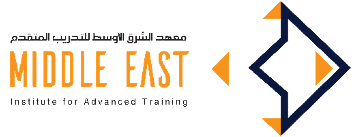Middle East Institute for Advanced TrainingCertification Body:
MOHERI

This course aims to provide participants with a thorough understanding of how to build a robust financial Model from start to finish.Calculations cover revenues, operating and maintenance costs, capitalExpenditure, depreciation, debt and equity financing and taxation, leading to the build-up of integrated Financial statements for the entity in question. The model is dynamic in nature, with the ability to run different scenarios and adjust the timing of key events.

During the course, participants also gain an insight into how to tailor the outputs of the model to end users, interpret the results and run sensitivities, as well as perform some degree of testing to reduce the incidence of modeling errors.

• Construct and examine the characteristics of distributions of returns
• Calculate the variance co-variance matrix and use it to select optional portfolios
• Test for market efficiency using simple tests
• Develop, construct and run an event study analysis of the abnormal returns
• Estimate betas and calculate a firm's cost of capital
• Calculate the value of an option using Black Scholes and the binomial model
• Use and develop spreadsheet based solutions to financial problems
• Introduction & Objectives of Financial Modeling
• Pre-requisites to Project Financial Modeling
• Key Structure for the Model Building
• Modeling best practices
• Inherent Tensions in Model Structuring
• Steps in creating Integrated Project Finance Model
• Project Finance Excel Shortcuts
• Model Inputs & DataValidation
• Financial Model processing & Outputs
• Model Grouping and Protection
• Understanding Financial Statements
• Profit & Loss(P&L) Statement
• Balance Sheet (B/S)
• Cash Flow Statement
• Financial Forecasting Modeling Framework
• Financial Merger, Acquisition & Disposal Modeling (PP& E, M & A)
• Capital Assets Pricing Model (CAPM)
• Debt & Financing Schedule • Building Cash Flow Forecasting Model
• Accountants,
• Financial analysts,
• Entrepreneurs,
• Students,
• Bank employees
Skills Empowered